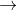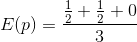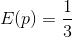##### Question

In: Statistics and Probability

# use the population {3,4,6} and assume that samples of size 2 are randomly selected, with replacement....

use the population {3,4,6} and assume that samples of size 2 are randomly selected, with replacement. a) for the population, find the proportion of odd numbers. b) construct a table for sampling distribution of the sample proportion of odd numbers. c) find the mean of sampling distribution of the sample proportion of odd numbers. d) is the sample proportion and unbiased estmator or. biased estimator of the population? Why?

## Solutions

##### Expert Solution

Given that,Use the population {3,4,6} and assume that samples of size 2 are randomly selected, with replacement.

(a).

For the population, find the proportion of odd numbers:From the given problem the population is {3,4,6}.

The proportion of odd numbers is,

In the data set {3,4,6} is odd number count is 1.

Therefore, the proportion of odd numbers is 1/3.

(b).

Construct a table for the sampling distribution of the sample proportion of odd numbers:The table representing the sampling distribution of the sample proportion of odd numbers is,

The data set is {3,4,6}

 Sample Proportion (P) (3,4) 1/2 (3,6) 1/2 (4,6) 0

(c).

Find the mean of the sampling distribution of the sample proportion of odd numbers:The mean of the sampling proportions,Thus, the required mean is 1/3.

(d).

Is the sample proportion and unbiased estimator [or] a biased estimator of the population:From the previous results,=P

Thus, the sample proportion is an unbiased estimator of the population proportion.

## Related Solutions

##### Use the population {3,4,6} and assume that samples of size 2 are randomly selected, with replacement....
Use the population {3,4,6} and assume that samples of size 2 are randomly selected, with replacement. ?) For the population, find the proportion of odd numbers ?) Construct a table for the sampling distribution of the sample proportions of odd numbers. ?) Find the mean of the sampling distribution of the sample proportion of odd numbers. ?) Is the sample proportion an unbiased estimator or a biased estimator of the population proportion? why?
##### Suppose samples of size 100 are drawn randomly from a population of size 1000 and the...
Suppose samples of size 100 are drawn randomly from a population of size 1000 and the population has a mean of 20 and a standard deviation of 5. What is the probability of observing a sample mean equal to or greater than 21?
##### 7. Use the population of {1, 3, 7}. Assume that the random samples of size n...
7. Use the population of {1, 3, 7}. Assume that the random samples of size n = 2 Construct a sampling distribution of the sample mean. After identifying the 9 different possible samples (with replacement), find the mean of each sample, then construct a table representing the sample the sampling distribution of the sample mean. In the table, combine values of the sample mean that are the same. (Hint: condense the table similar to examples presented in class.) a. Sample...
##### Assume that a population of size 5 specifies that all possible samples of size "3" are...
Assume that a population of size 5 specifies that all possible samples of size "3" are extracted without repetition. Values: 2,500.00 2,650.00 2,790.00 3,125.00 3,200.00 1) Show that the expected value of the standard sampling error is greater than the standard deviation of the population. 2) Calculate the confidence interval of the sample means with a significance level of 80% and indicate which samples are not representative and develop an analysis. 3) Select the non-representative samples and calculate the mean...
##### Assume that a population of size 5 specifies that all possible samples of size "3" are...
Assume that a population of size 5 specifies that all possible samples of size "3" are extracted without repetition. Values: 2,500.00 2,650.00 2,790.00 3,125.00 3,200.00 1. Show that the expected value of the standard sampling error is greater than the standard deviation of the universe. 2. Select a sample and calculate the mean of the universe mean with a confidence level of 84% and develop an analysis.
##### Assume that the samples are independent and that they have been randomly selected. Construct a​ 90%...
Assume that the samples are independent and that they have been randomly selected. Construct a​ 90% confidence interval for the difference between population proportions p1-p2. Round to three decimal places. x1=12​, n1=45 and x2=21​, n2=51 A. -0.301 <p1-p2< 0.011 B. 0.453 <p1−p2 < 0.079 C. 0.109 <p1−p2< 0.425 D. 0.081 <p1−p2< 0.453
##### Assume a population of 1,2 and 12. assume the sample size of size n = 2...
Assume a population of 1,2 and 12. assume the sample size of size n = 2 are randomly selected with replacement from the population. listed below are the nine different sample. complete parts a through d below 1,1 1,2 1,12, 2,1 2,2 2,12 12,1 12,2 12,12 a) find the value of the population standard deviation b) find the standard deviation of each of the nine samples the summarize the sampling distribution of the standard deviation in the format of a...
##### Random samples of size n = 90 were selected from a binomial population with p =...
Random samples of size n = 90 were selected from a binomial population with p = 0.8. Use the normal distribution to approximate the following probability. (Round your answer to four decimal places.) P(p̂ > 0.78) =
##### Random samples of size n = 60 were selected from a binomial population with p =...
Random samples of size n = 60 were selected from a binomial population with p = 0.2. Use the normal distribution to approximate the following probabilities. (Round your answers to four decimal places.) (a)     P(p̂ ≤ 0.22) = (b)     P(0.18 ≤ p̂ ≤ 0.22) =
##### Two samples, one of size 13 and the second of size 18, are selected to test...
Two samples, one of size 13 and the second of size 18, are selected to test the difference between two population means and σ is unknown. Which distribution should be used for this test? What is the critical value for a 10% level of significance for a right tail test?